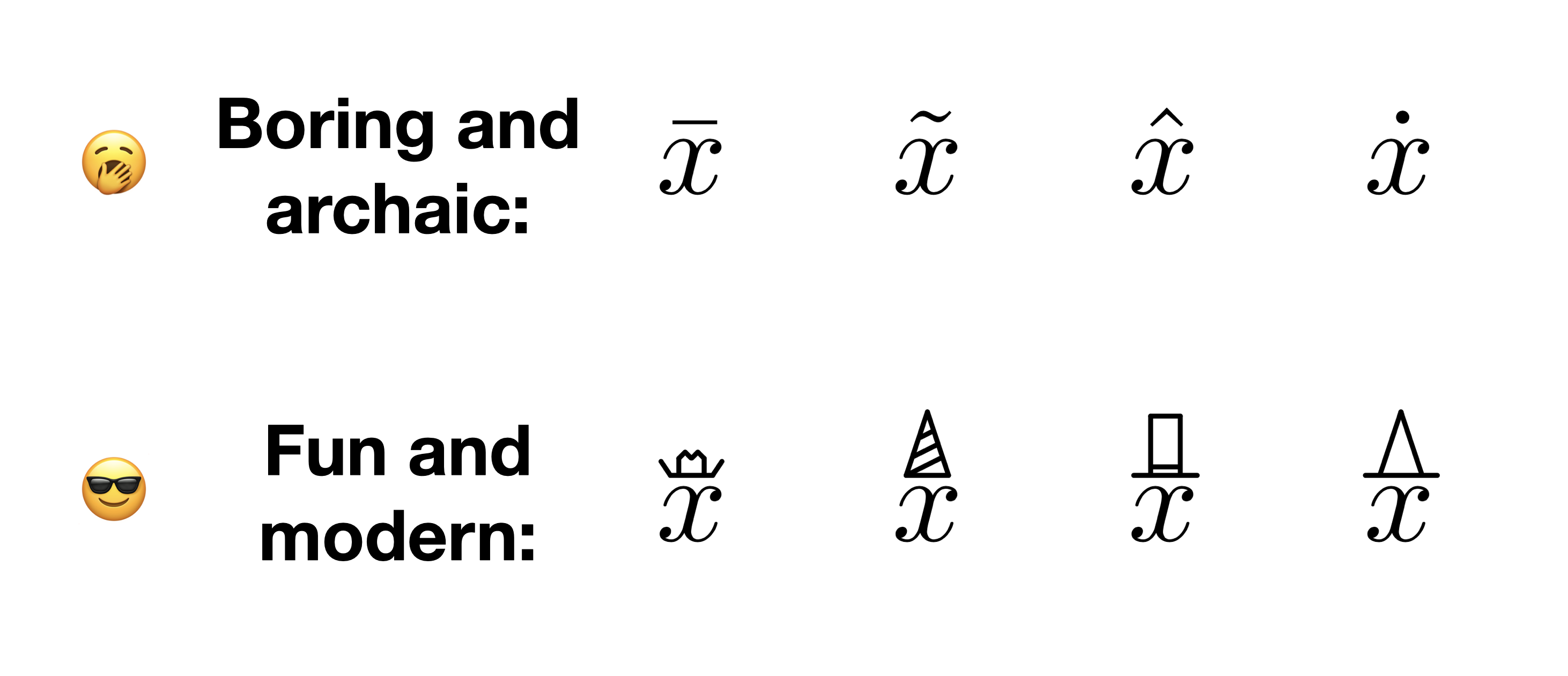# Modernizing Hat Operators in Latex

Are you tired of the same old hat operators? Does \bar{} or \hat{} make you want to yawn? Even worse, is the boredom induced by these markers causing you to fall behind in classes?

I say out with the old, in with the new, so check out these hats. Engaging enough to get you that A, subtle enough to let the math do the talking.BibTeX entry for post:

@misc{belsten2023hats,
author = {Belsten, Alexander},
title = {Modernizing Hat Operators in Latex},
howpublished = "\url{https://belsten.github.io/blog/latex-hats/}",
date = {2023-03-30}
}


How to reproduce:

\documentclass{article}
\usepackage{pict2e}

\DeclareRobustCommand{\howdyhat}{
\begingroup\setlength{\unitlength}{\fontcharht\fontA}
\begin{picture}(.5,1)
\roundcap
\put(-0,-.15){\line(-1,1.5){0.11}}
\put(0.5,-.15){\line(1,1.5){0.11}}
\put(-0,-.15){\line(1,0){0.5}}
\put(0.1,-.15){\line(0,1){0.2}}
\put(0.4,-.15){\line(0,1){0.2}}
\put(0.1,0.05){\line(1,1){0.075}}
\put(0.4,0.05){\line(-1,1){0.075}}
\put(0.18,0.12){\line(1,-1){0.065}}
\put(0.32,0.12){\line(-1,-1){0.065}}
\end{picture}
\endgroup
}

\DeclareRobustCommand{\partyhat}{
\begingroup\setlength{\unitlength}{\fontcharht\fontA}
\begin{picture}(.5,1)
\roundcap
\put(-0,-.15){\line(1,3){0.25}}
\put(-0,-.15){\line(1,0){0.5}}
\put(0.5,-.15){\line(-1,3){0.25}}
\put(-0,-.15){\line(1,.5){0.4}}
\put(0.09,0.075){\line(1,.5){0.27}}
\put(0.17,0.3){\line(1,.5){0.13}}
\end{picture}
\endgroup
}

\DeclareRobustCommand{\abehat}{
\begingroup\setlength{\unitlength}{\fontcharht\fontA}
\begin{picture}(.5,1)
\roundcap
\put(-0.1,-.15){\line(1,0){0.75}}
\put(0.12,-.05){\line(1,0){0.32}}
\put(0.1,-.15){\line(0,1){0.7}}
\put(0.45,-.15){\line(0,1){0.7}}
\put(0.1,0.55){\line(1,0){0.35}}
\end{picture}
\endgroup
}

\DeclareRobustCommand{\witchhat}{
\begingroup\setlength{\unitlength}{\fontcharht\fontA}
\begin{picture}(.5,1)
\roundcap
\put(-0.15,-.15){\line(1,0){0.85}}
\put(0.02,-.15){\line(1,3){0.25}}
\put(0.52,-.15){\line(-1,3){0.25}}
\end{picture}
\endgroup
}

\newcommand\popacaponit{\mathrel{\stackrel{\makebox[0pt]{\mbox{$#2$}}}{#1}}}
\newcommand\howdy{\popacaponit{#1}{\howdyhat}}
\newcommand\party{\popacaponit{#1}{\partyhat}}
\newcommand\abe{\popacaponit{#1}{\abehat}}
\newcommand\witch{\popacaponit{#1}{\witchhat}}

\begin{document}
\begin{equation}
\howdy{x} \ \ \ \ \party{x} \ \ \ \ \abe{x} \ \ \ \ \witch{x}
\end{equation}
\end{document}


Updated: